﻿ 基于偏微分方程的模型光滑处理在偏移成像中的应用
 地球物理学报2018, Vol. 61Issue (11): 4558-4567PDF

1. 吉林大学地探学院波动理论与成像技术实验室, 长春 130026;
2. 吉林交通职业技术学院, 长春 130012

The applications of model smoothing based on partial differential equation in migration imaging
HAN FuXing1, SUN JianGuo1, WANG Kun2
1. Theory of Ministry for Land and Resources, College for Geoexploration Science Technology, Jilin University, Changchun 130026, China;
2. Jilin Communications Polytechnic, Changchun 130012, China
Abstract: In view of the problem of velocity model smoothing in ray type migration imaging, by reference to partial differential equation method in digital image processing, and based on the energy functional, we derived the partial differential equations based on velocity model, using the variational method to achieve velocity model smoothing in ray type migration imaging. Because the partial differential equations have the characteristics of linear superposition, model solution uniqueness and local features retention, therefore, the application of the algorithm can be very good to keep edge characteristics and the characteristics smoothing of velocity model, without breaking the spatial structure of the original velocity model. By calculating the spatial distribution of velocity, the travel time, and the ray paths of the seismic waves on the original and smoothed velocity model, it can be concluded that the smoothing of the velocity model using PDE method is good for keeping the spatial structure of the original model, the results of migration imaging also prove the practicability of this method.
Keywords: Partial differential equation    Model smoothing    Convolution operator    Migration imaging
0 引言

1 偏微分方程模型光滑处理算法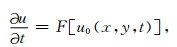(1)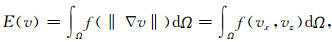(2)

Ω为速度模型的大小，f(·)≥0为递增函数.在速度模型横向和纵向变化比较剧烈的区域，速度梯度的模就越大，因此，要达到图像平滑的目的就要最小化能量函数E(v).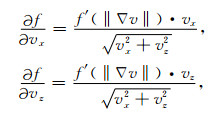(3)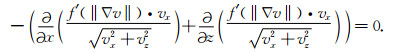(4)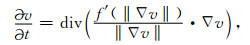(5)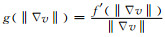，则上式变形为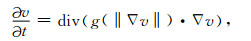(6)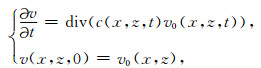(7)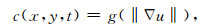(8)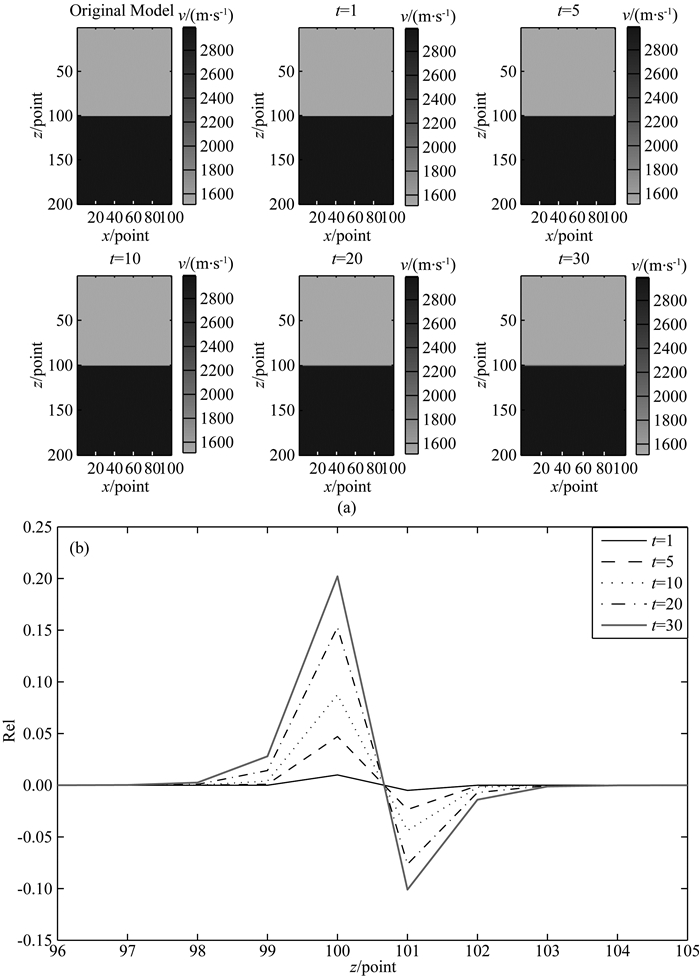图 1 PDE算法对速度模型的光滑处理 (a)两层原始速度模型及对分界面光滑处理后的两层速度模型; (b)不同迭代次数处理后的速度模型与原始速度模型在分界面处速度的相对误差分布. Fig. 1 Smooth processing velocity model using Partial Differential Equation algorithm (a) The two layer original and smoothed interface velocity model using PDE algorithm; (b) Relative error of the original and after Different Iteration Smoothing velocity model.

2 计算测试 2.1 速度模型空间结构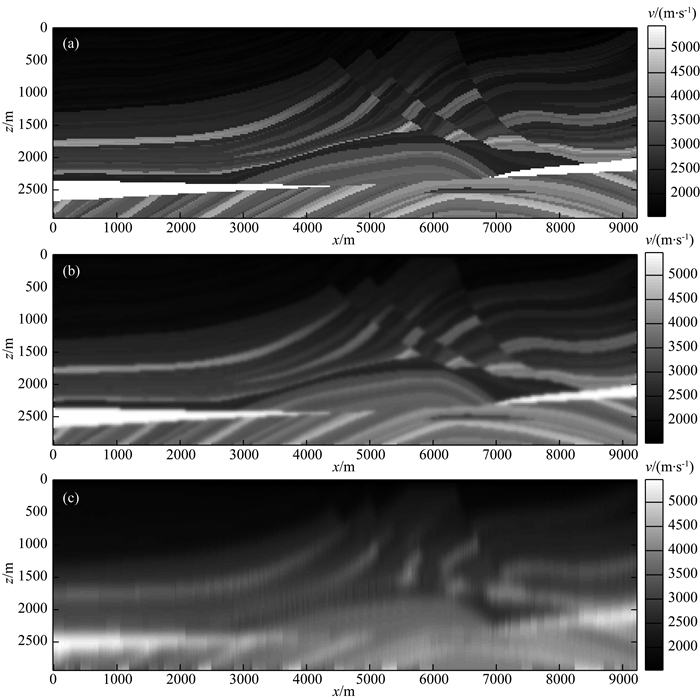图 2 Marmousi原始速度模型以及光滑处理后的速度模型 (a)原始Marmousi速度模型; (b)应用PDE光滑处理迭代30次后的速度模型; (c)卷积算子光滑30次后的速度模型. Fig. 2 The original and smoothing velocity model using PDE algorithm of Marmousi (a) Original velocity model of Marmousi; (b) The velocity model of Smoothing iteration 30 times using Partial Differential Equation algorithm; (c) The velocity model of Smoothing 30 times using Convolution Operator.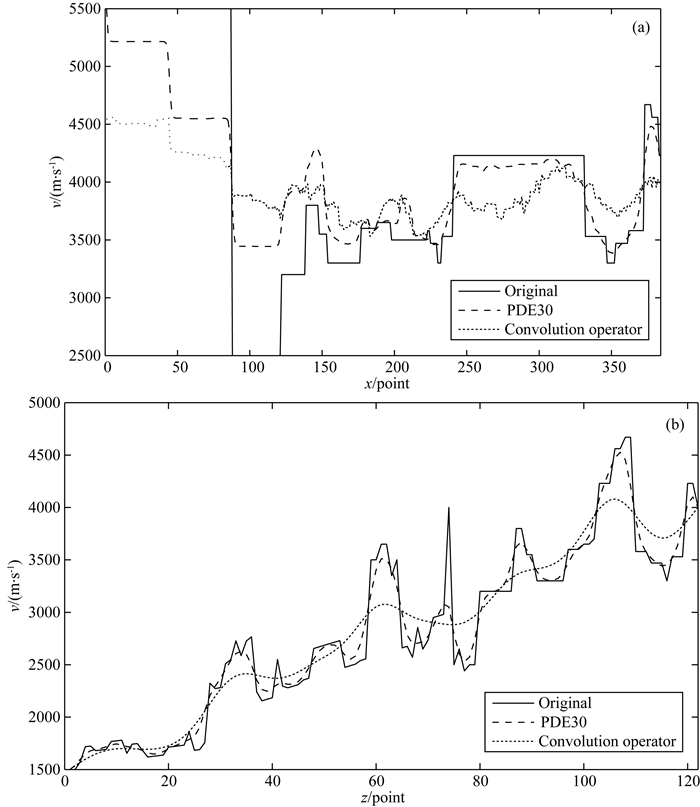图 3 原始Marmousi速度模型以及光滑处理后速度模型在x方向和z方向上的空间结构对比 (a) z=100点位，x方向所有点的速度值对比; (b) x=192点位，z方向上的所有速度值对比. Fig. 3 Contrast spatial structure of original and smoothing velocity model using PDE algorithm of Marmousi velocity model (a) z=100 point, the velocity difference of all points in the x direction; (b) x=100 point, the velocity difference of all points in the z direction.

2.2 走时误差分析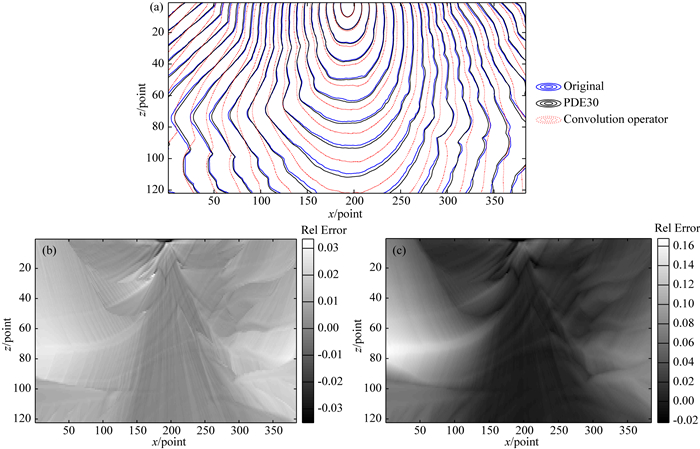图 4 光滑处理前后Marmousi速度模型上地震波走时以及相对误差 (a)原始Marmousi速度模型和光滑处理后的速度模型走时对比; (b) PDE算法光滑处理后的走时相对误差; (c)卷积算子光滑处理后的走时相对误差. Fig. 4 Seismic travel time and relative error distribution of Marmousi model (a) Travel time distribution of original and smoothing velocity model; (b) Traveling Time Relative Error using PDE algorithm; (c) Traveling Time Relative Error using Convolution Operator.

2.3 射线路径对比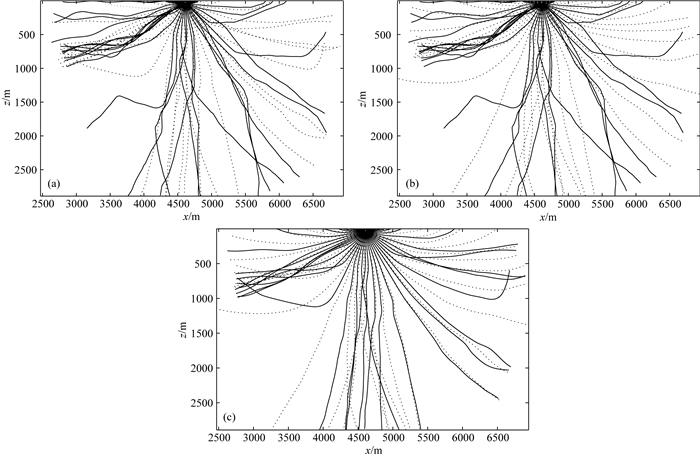图 5 原始Marmousi速度模型、PDE算法及卷积算子光滑处理后模型上的射线路径对比 (a)原始速度模型及PDE算法光滑处理后的射线路径对比(实线为原始速度模型); (b)原始速度模型及卷积算子光滑处理后的射线路径对比(实线为原始速度模型); (c) PDE算法以及卷积算子光滑处理后的射线路径对比(实线为PDE光滑后的模型射线路径, 虚线为卷积算子). Fig. 5 The difference ray paths of original velocity model and using PDE Algorithm Convolution Operator (a) The difference ray paths of original and smoothing velocity model using PDE Algorithm(Solid line is original velocity model); (b) The difference ray paths of original and smoothing velocity model using Convolution Operator (Solid line is original velocity model); (c) The difference ray paths of velocity model using PDE Algorithm and Convolution Operator (Solid line is PDE Algorithm).

3 在偏移成像中的应用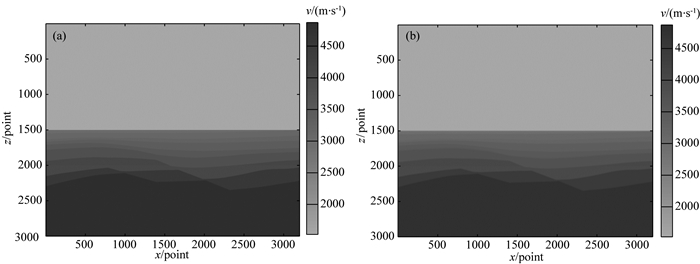图 6 原始大水深速度模型以及应用PDE光滑处理后的速度模型 (a)原始速度模型; (b) PDE光滑处理后的速度模型(t=10). Fig. 6 The original complex seabed model and smoothing velocity model using PDE algorithm (t=10) (a) The original complex seabed velocity model; (b) Smoothing velocity model using PDE algorithm (t=10).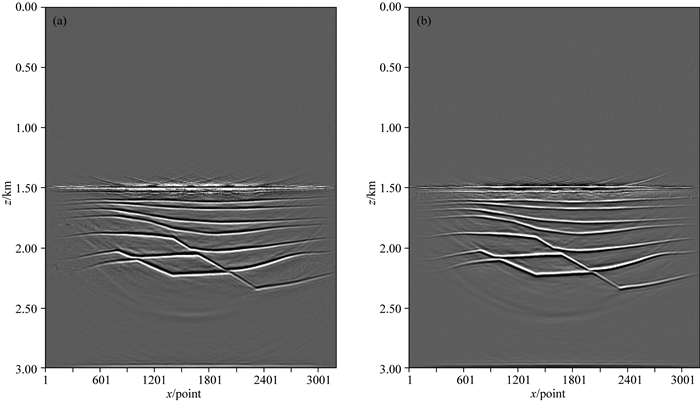图 7 原始速度模型以及光滑处理后的速度模型上的偏移成像结果 (a)原始速度模型的偏移结果; (b) PDE算法光滑处理后模型的偏移结果. Fig. 7 The migration imaging results of the original and smoothing using PDE algorithm (t=10) (a) The migration imaging results of the original velocity model; (b) The migration imaging results of smoothing velocity model using PDE algorithm (t=10).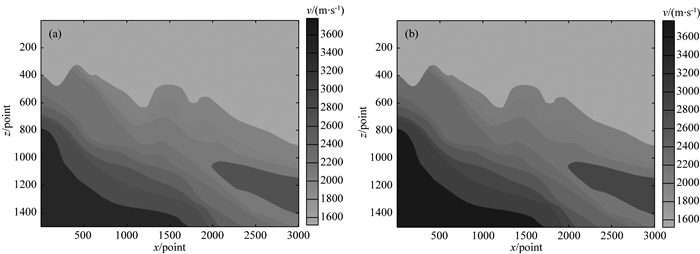图 8 原始速度模型以及应用PDE光滑处理30次后的速度模型 (a)原始崎岖海底大陡坡速度模型; (b) PDE光滑处理30次后崎岖海底大陡坡速度模型. Fig. 8 The original and smoothing velocity model using PDE algorithm (t=30) (a) The original rough and steep slope rugged seabed velocity model; (b) The rough and steep slope rugged seabed velocity model smoothed using PDE algorithm (t=30).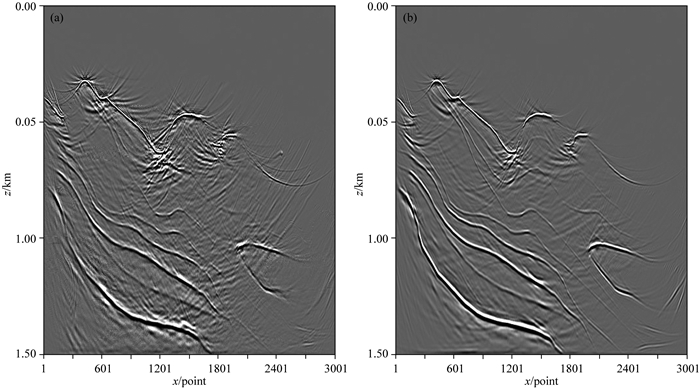图 9 原始崎岖海底大陡坡速度模型以及光滑处理后的速度模型上的偏移成像结果 (a)原始崎岖海底大陡坡速度模型偏移成像结果; (b) PDE光滑处理30次后崎岖海底大陡坡速度模型偏移结果. Fig. 9 The migration imaging results of the original rough and steep slope rugged seabed velocity model and smoothed using PDE algorithm (t=30) (a) The migration imaging results of the original rough and steep slope rugged seabed velocity model; (b) The migration imaging results of smoothing rough and steep slope rugged seabed velocity model using PDE algorithm (t=30).

4 结论

References
 Alvarez L, Lions P L, Morel J M. 1992. Image selective smoothing and edge detection by nonlinear diffusion.Ⅱ. SIAM Journal on Numerical Analysis, 29(3): 845-866. DOI:10.1137/0729052 Carmona R A, Zhong S F. 1998. Adaptive smoothing respecting feature directions. IEEE Transactions on Image Processing, 7(3): 353-358. DOI:10.1109/83.661185 Caselles V, Morel J. 1998. Introduction to the special issue on partial differential equations and geometry-driven diffusion in image processing and analysis. IEEE Transactions on Image Processing, 7(3): 269-273. DOI:10.1109/TIP.1998.661176 Catte F, Coll T, Lions P L, et al. 1992. Image selective smoothing and edge detection by nonlinear diffusion.Ⅰ. SIAM Journal on Numerical Analysis, 29(3): 182-193. Ettrich N, Gajewski D. 1996. Wave front construction in smooth media for prestack depth migration. Pure and Applied Geophysics, 148(3-4): 481-502. DOI:10.1007/BF00874576 Gabor D. 1965. Information theory in electron microscopy. Laboratory Investigation:A Journal of Technical Methods and Pathology, 14: 801-807. Gray S H. 2000. Velocity smoothing for depth migration: how much is too much?.//70th Ann. Internat Mtg., Soc. Expi. Geophys.. Expanded Abstracts. Han F X. 2009. On some computational problems in wavefront construction method [Ph. D. thesis] (in Chinese). Changchun: Jilin University. Han F X, Sun J G, Wang K. 2011. Analysis of velocity model smoothing. Journal of Jilin University (Earth Science Edition) (in Chinese), 41(5): 1610-1616, 1645. Hummel R. 1987. Representations based on zero-crossing in scale-space. Readings in Computer Vision, 753-758, Published by Courant Institute of Mathematical Sciences, New York University, in New York. Kass M, Wiltkin A, Terzopoulos D. 1987. Snakes:active contour models. International Journal of Computer Vision, 1(4): 321-331. Koenderink J J. 1984. The structure of image. Biological Cybernetics, 50(5): 363-370. DOI:10.1007/BF00336961 Lailly P, Sinoquet D. 1996. Smooth velocity models in reflection tomography for imaging complex geological structures. Geophysical Journal International, 124(2): 349-362. DOI:10.1111/gji.1996.124.issue-2 Larner K, Pacheco C. 2005. Velocity smoothing before depth migration:Does it help or hurt?. Seg Technical Program Expanded Abstracts, 24(1): 1970. Perona P, Malik J. 1990. Scale-space and edge detection usinganisotropic diffusion. IEEE Transactions on Pattern Analysis and Machine Intelligence, 12(7): 629-639. DOI:10.1109/34.56205 韩复兴. 2009.论波前构建法中的几个计算问题[博士论文].长春: 吉林大学. 韩复兴, 孙建国, 王坤. 2011. 速度模型的光滑处理分析. 吉林大学学报(地球科学版), 41(5): 1610-1616, 1645.